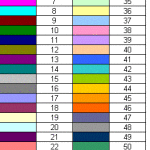# Excel VBA set print areaThis Excel VBA tutorial explains how to set print area, page width, Print Title, Orientation in Excel VBA. Excel VBA set print area To set Print Area in Excel spreadsheet, first select (highlight) Cells that we want to set as Print Area, and then navigate to ribbon Page Layout > Print Area > Set Print Area To set Print Area in Excel VBA, we have to use PageSetup.PrintArea Property. For example,  in order to set Print Area A1:N21, we can write Public Sub printArea()     Active...
More

# Excel VBA freeze panes using FreezePanes propertyThis Excel tutorial explains how to freeze panes in Excel spreadsheet and freeze panes in Excel VBA using FreezePanes property. Freeze Panes in Excel spreadsheet To explain how to freeze panes in Excel VBA, first I have to explain how to freeze panes in Excel spreadsheet. Freeze Row The most common kind of freeze panes is to freeze the first row as it contains the header of the column. So that when you scroll down the spreadsheet, you can still see the header in row 1. Highlight row 2, na...
More

# Excel VBA Named Range PropertiesThis Excel VBA tutorial explains some common Properties of Name Object (Named Range), and demonstrate how to replace named Range with formula. You may also want to read: Excel find all external links and broken links in workbook Excel VBA Named Range Properties In Excel spreadsheet, we can define Named Range under Name Manager (Formulas > Name Manager) In the below screenshot, I have defined a Named Range called testNameRng, which refers to Cell A1 in Worksheet1. Currently A1 conta...
More

# Excel VBA Application WorksheetFunction PropertyThis Excel VBA tutorial explains how to use Application WorksheetFunction Property in Excel VBA. Excel VBA Application WorksheetFunction Property Excel VBA Functions and Excel Worksheet Functions are slightly different. Sometimes even though the Function names and syntax are the same, the results are different. There are many nifty Worksheets Functions but unfortunately they are not present in VBA, and vice versa. In order to use Worksheet Function in VBA, use Application WorksheetFunction ...
MoreMore

# Excel VBA usedRange Property and reset usedRangeThis tutorial explains how to use Excel VBA usedRange Property to find the last used row and column number, and reset usedRange. You may also want to read: Excel VBA custom Function last row and last column Excel delete blank rows and blank cells before import into Access Excel VBA UsedRange Property Excel VBA UsedRange is a worksheet Property, it returns the area Range bounded by first used cell and last used cell. "Used Cell" is defined as Cell containing formula, formatting, value th...
More

# Excel VBA Application Calculation PropertyThis tutorial explains Excel Application Calculation Property and difference among xlCalculationAutomatic, xlCalculationManual, xlCalculationsemiautomatic Excel VBA Application Calculation Property In Excel worksheet, there are three options under Workbook Calculation. The options in Workbook Calculation uses Calculation Property in Excel VBA, which means if you execute the corresponding VBA code, the Excel options change accordingly. Excel Option Description VBA Automatic...
More

# VBA Excel ColorIndex PropertyThis tutorial explains how to use VBA Excel colorIndex Property to set color and get color from Cell or Shape, meaning of -4142, -4105, xlNone, xlAutomatic What does VBA Excel ColorIndex Property do? VBA Excel ColorIndex Property is to set color or get color for Objects like Cell color and Shape color. ColorIndex offers 56 basic colors plus the following special numbers. ColorIndex Value Explanation -4142 / xlColorIndexNone / xlNone No fill -4105 / xlColorIndexAutomatic / xl...
More

# VBA Excel RGB Property and get RGB ColorWhat does VBA Excel RGB Property do? VBA Excel RGB Property is a color Property of Objects, commonly used for Cell color or Shape color. "RGB" stands for Red Green Blue, which are known as three additive primary colors, which can be combined to produce other colors. For example, purple is a mix of blue and red.  In RGB Property, you need to give a value (from 0 to 255) for each color in order to mix a new color. For example, Red = 255 means the brightness of color red is 100%. If you ...
More

# Excel VBA Formula Property of RangeThis Excel VBA tutorial explains how to use Formula Property in Excel VBA. Formula Property in Excel VBA There are two functions for Formula Property: 1. To set formula in a Range, like the way you type a formula in worksheet 2. To retrieve formula from a Range Syntax of  Formula Property To set a formula: Range.Formula = "=your formula" To retrieve a formula: variableName = Range.Formula Remarks You can also set a formula using Value Property Range.Value = "=your formula" ...
More